## Understanding of discrete signals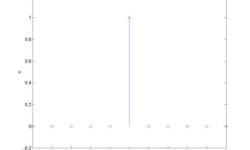Discrete signals can be generated by software or obtained from the real-world through ADC. Discrete signals are sampled from analog signals. So you get samples in fixed time intervals. The discrete signal is a sequence of numbers. The element number n of the sequence is marked as x(n). The most common number rows: Unit sample sequence You can describe it in Matlab like

## Physiological system modeling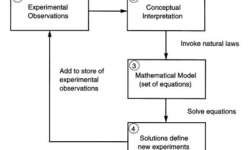Most physical and chemical experiments can be described with a finite number of rules. In physiological processes, everything can be tied to the laws of nature. A good model can be a guideline for new experiments. Usually, the model and experiment have an iterative connection. To understand how to investigate any material, there is a model needed (block 1). First, we need an abstract understanding of what we are investigating (block 2). Abstracts are transformed into mathematical models (block 3). There we can use formulas and solve them using the computer. The results you get shows, what experiments have to be done, and what to expect from them. This loop system is resistive to irregularities in the mathematical model. In other words, this is the purifier of experiments and models.

## CMOS and TTL compliance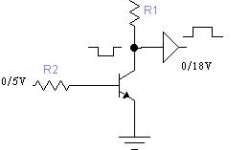There was a time when all IC’s were only TTL type. They were marked as 74LS or 74ALS. But then there came CMOS types of semiconductors. They are usually marked CDxx. And lately, there appeared combined semiconductors that are compliant with both types. They usually are marked as 74HC or 74HCT.TTL is faster than CMOS, but they sink more power. They are powered from a 5V source. To diminish the power consumption, the CMOS was developed. They can work with supplied voltages in the range of 3 to 15V. The main disadvantage is that they are static sensitive. So you always have to be grounded when working with CMOS.Now both types of semiconductors are widely used. Sometimes there is a dilemma to connect TTL to CMOS. You cannot connect TTL directly to CMOS as there are different supply voltages. So it would help if you had some compliance circuit. The simplest can be bipolar transistor cascade. Just remember that such a cascade inverts the signal, so you might need to put an inverter in front of CMOS. Another reminder – never leave TTL or CMOS free pins hanging. Jus connect them through a resistor to VCC or GND.

## Skin lesion boundary tracing algorithm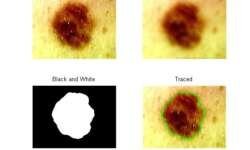I found Matlab to be a convenient tool that easily traces the boundaries of objects in a picture. So I adopted it to skin lesions. This can be used to automatically detect skin irregularities and calculate lesion properties like the asymmetry of shape, or border irregularities, which can help detect melanoma. There are numerous investigations done, so I only put a few examples of how it looks. I will give you my source code so that you can try it on your own. Look at my results: 1) And it also finds the center of mass:

## Skin imaging methods for melanoma diagnosis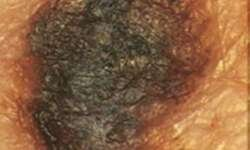There are many skin image capture methodologies developed and used. Here is a short review of them: Dermatoscopic photography The deepest layer of skin can be reached – Papillary dermis Resolution – depends on the optical system View of skin – Horizontal The main disadvantage is reflections of light from skin surface – stratum cornea.

## Polarizer test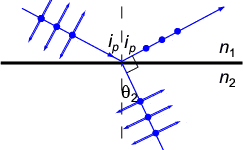I used a simple lamp directed to the glossy table surface. One polarizer is in front of the lamp, and the other is in front of a digital camera lens. Both polarizes perpendicularly oriented to each other. How does this work? A theory about an angle on which the incident polarized electromagnetic waves turn reflects from the surface with a polarization plane turned in 90 degrees. When light going through the polarizer towards the surface, the light is polarized in one direction, and when it reflects from the surface, it is turned by 90 degrees. Another perpendicular polarizer filters those waves in front of the lens.

## Removing 60Hz from ECG using digital band stop filter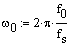Let’s make a filter, which filters off the 60Hz frequency from the ECG signal. As we know, the American power supply is 60Hz. This is a common noise in biomedical signals while the industrial power supply powers them. This type of noise can be defined easily and can be filtered as parameters of noise are known.Here is one example of how to implement an FIR filter using mathematical tools, like Matlab. This can be done using a microcontroller, like ARM or even AVR, because the frequencies are up to 1 kHz.Initial conditions: f0=60Hz – power supply frequency;fs=500Hz – sampling rate;frequencies who define complex zeros: we get w0=0.754;Positions of complex zeros: Zeros and poles in z plane System Function From it we can calculate filter coefficients: And filter coefficients: b3 = 1; b2 = -2cos(w0); b1 = 1 Also we know that: And here we get filter characteristics: We have a band stop filter at 60Hz, and its jam at 60Hz is -300dB. Bellow is a filter structure: Now using this filter we can filter ECG signal: As you can see, this is a simple FIR filter. In other words, there is nothing more than an average function that doesn’t need…

## Imaging system with four different wavelengths obtained from LEDs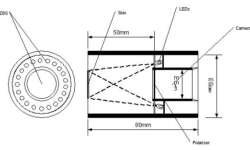To evaluate skin pigmentation in different skin layers, a special light adapter is needed to take multispectral pictures of the skin. As there are different optical properties of skin pigments, four different light sources have been chosen. blue λ= 470 nm – highly absorbed by epidermal melanin green λ= 576 nm – hemoglobin peak red  λ= 660nm – epidermal-dermal boundary IR λ= 865 nm – low absorption, sensitive to scattering to measure papillary dermis thickness. There was a lighting source for the “Nikon Coolpix E3100” digital camera developed to take multispectral images of skin. Making an adapter The drawing of  lighting adapter The lightning adapter isn’t tough to build. It would help if you made a circular PCB and solder LEDs with protective resistors. The PCB image: